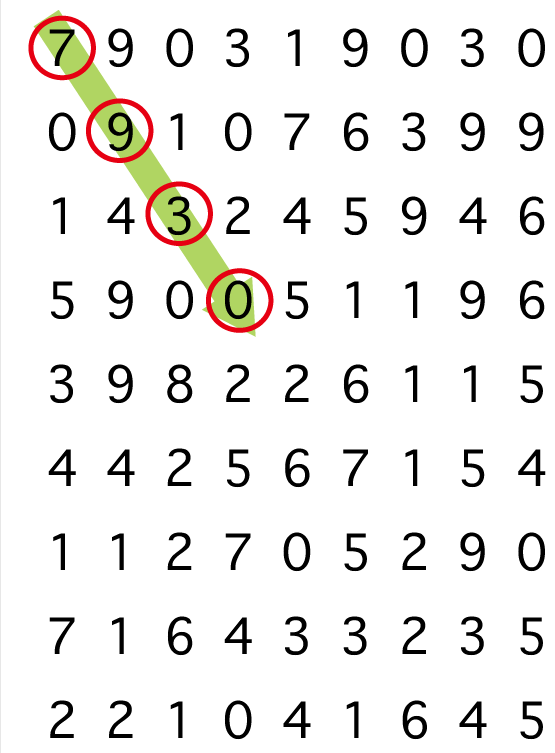• 原方向是上下左右时
• 将原方向180°调转
• 原方向是斜向时
• 在对角线上时：将原方向180°调转
• 遇到左右边界时：上下方向不变，左右方向调转
• 遇到上下边界时：左右方向不变，上下方向调转

• 共10行输入
• 第1行，整数x代表列(1≤x≤9)，整数y代表行(1≤y≤9)，W表示方向
• W是R、L、U、D、RU、RD、LU、LD的其中一种
• R：右方向
• L：左方向
• U：上方向
• D：下方向
• RU：右上方向
• RD：右下方向
• LU：左上方向
• LD：左下方向
• 第2行到第9行是密码表中的整数C[i][j](1≦i,j≦9)
• i和j表示第i行第j列数字C[i][j]
• Cij的范围是0到9

scanf("%1d",&mp[i][j]);

#include<map>
#include<string>
map<string,int>h1,h2;
int main(){  　　h1["R"]=0;h2["R"]=1;h1["L"]=0;h2["L"]=-1;h1["U"]=-1;h2["U"]=0;   　　h1["D"]=1;h2["D"]=0;h1["RU"]=-1;h2["RU"]=1;h1["LU"]=-1;h2["LU"]=-1;　　h1["RD"]=1;h2["RD"]=1;h1["LD"]=1;h2["LD"]=-1;
}

 1 bool chujie(){
2     if(x<=0||x>=10||y<=0||y>=10){
3         return false;
4     }else{
5         return true;
6     }
7 }
8 int main(){
9     for(int i=1;i<=4;i++){
10         cout<<mp[x][y];
11         x+=h1[w];y+=h2[w];
12         if(chujie()==false){
13             zhuan();
14         }
15     }
16     cout<<endl;
17     return 0;
18 }

 1 #include<iostream>
2 #include<map>
3 #include<cstdio>
4 #include<string>
5 using namespace std;
6 int x,y;
7 string w;
8 int mp;
9 map<string,int>h1,h2;
10 bool chujie(){
11     if(x<=0||x>=10||y<=0||y>=10){
12         return false;
13     }else{
14         return true;
15     }
16 }
17 void zhuan(){//改变方向
18     if(w=="U"){//原方向向上
19         x+=2;w="D";
20     }else if(w=="D"){
21         x-=2;w="U";
22     }else if(w=="L"){
23         y+=2;w="R";
24     }else if(w=="R"){
25         y-=2;w="L";
26     }else{
27         if(w=="LU"&&x==0&&y==0){//先处理对角线
28             x+=2;y+=2;w="RD";
29         }else if(w=="LD"&&x==10&&y==0){
30             x-=2;y+=2;w="RU";
31         }else if(w=="RU"&&x==0&&y==10){
32             x+=2;y-=2;w="LD";
33         }else if(w=="RD"&&x==10&&y==10){
34             x-=2;y-=2;w="LU";
35         }else if(y==10){//撞右边界
36             if(w=="RU"){
37                 y-=2;w="LU";
38             }else if(w=="RD"){
39                 y-=2;w="LD";
40             }
41         }else if(y==0){//撞左边界
42             if(w=="LU"){
43                 y+=2;w="RU";
44             }else if(w=="LD"){
45                 y+=2;w="RD";
46             }
47         }else if(x==0){//撞上边界
48             if(w=="LU"){
49                 x+=2;w="LD";
50             }else if(w=="RU"){
51                 x+=2;w="RD";
52             }
53         }else if(x==10){//撞下边界
54             if(w=="RD"){
55                 x-=2;w="RU";
56             }else if(w=="LD"){
57                 x-=2;w="LU";
58             }
59         }
60     }
61 }
62 int main(){
63     cin>>y>>x>>w;//x是行，y是列
64     for(int i=1;i<=9;i++){
65         for(int j=1;j<=9;j++){
66             scanf("%1d",&mp[i][j]);
67         }
68     }
69     h1["R"]=0;h2["R"]=1;h1["L"]=0;h2["L"]=-1;h1["U"]=-1;h2["U"]=0;
70     h1["D"]=1;h2["D"]=0;h1["RU"]=-1;h2["RU"]=1;h1["LU"]=-1;h2["LU"]=-1;
71     h1["RD"]=1;h2["RD"]=1;h1["LD"]=1;h2["LD"]=-1;
72     for(int i=1;i<=4;i++){
73         cout<<mp[x][y];
74         x+=h1[w];y+=h2[w];
75         if(chujie()==false){
76             zhuan();
77         }
78     }
79     cout<<endl;
80     return 0;
81 }

## AT212 P-CASカードと高橋君的更多相关文章

1. 高橋君とホテル / Tak and Hotels

高橋君とホテル / Tak and Hotels Time limit : 3sec / Stack limit : 256MB / Memory limit : 256MB Score : 700  ...

2. 高橋君とカード / Tak and Cards

高橋君とカード / Tak and Cards Time limit : 2sec / Stack limit : 256MB / Memory limit : 256MB Score : 300 p ...

3. AT987 高橋君

AT987 高橋君 给出 $$n,\ k$$ ,求 $$\displaystyle\sum_{i=0}^kC_n^k$$ , $$T$$ 次询问 $$T\leq10^5,\ 0\leq k\leq n ... 4. 高橋君とカード / Tak and Cards AtCoder - 2037 （DP） Problem Statement Tak has N cards. On the i-th (1≤i≤N) card is written an integer xi. He is selectin ... 5. AtCoder Beginner Contest 044 C - 高橋君とカード / Tak and Cards 题目链接:http://abc044.contest.atcoder.jp/tasks/arc060_a Time limit : 2sec / Memory limit : 256MB Score ... 6. AtCoder Beginner Contest 044 A - 高橋君とホテルイージー / Tak and Hotels (ABC Edit) Time limit : 2sec / Memory limit : 256MB Score : 100 points Problem Statement There is a hotel with ... 7. 【AT987】高橋君 题目 成爷爷一眼秒,\(tql!!!$$ 多组询问,求 $\sum_{i=0}^kC_{n}^i$ 发现$$k<=n$$啊,于是我们可以把一组询问抽象成一个区间$$[k,n]$$ 左指针的 ...

8. AtCoder D - 高橋君と見えざる手 / An Invisible Hand 简单思维题

9. AtCoder Beginner Contest 022 A.Best Body 水题

Best Body Time Limit: 20 Sec  Memory Limit: 256 MB 题目连接 http://abc022.contest.atcoder.jp/tasks/abc02 ...

10. AtCoder-arc060 (题解)

A - 高橋君とカード / Tak and Cards (DP) 题目链接 题目大意: 有 $$n$$ 个数字,要求取出一些数字,使得它们的平均数恰好为 $$x$$ ,问有几种取法. 大致思路: 只要 ...

## 随机推荐

1. unity 2D 物体跟随鼠标旋转 移动

using System.Collections; using System.Collections.Generic; using UnityEngine; public class FollowMo ...

2. Android studio java文件显示j爆红

今天在android studio打开一个原来的工程,此工程是很久以前使用eclipse创建的,在android studio下有些问题需要解决. 1.设置project的jdk, 2.设置modul ...

3. /usr/bin/install: cannot create regular file `/usr/local/jpeg6/include/jconfig.h'

出现下列异常: /usr/bin/install -c -m 644 jconfig.h /usr/local/jpeg6/include/jconfig.h /usr/bin/install: ca ...

4. JavaScript逗号运算符的用法

var a = 3, b b = (a++, a) 与 var a = 3, b b = a++ 区别

5. 发现C++程序中未释放的内存空间

本篇先后介绍在windows中使用visual studio定位未释放的内存.在linux中使用valgrind定位未释放的内存. Windows+Visual Studio 2015 (企业版) 准 ...

6. Python学习笔记文件读写之生成随机的测试试卷文件

随笔记录方便自己和同路人查阅. #------------------------------------------------我是可耻的分割线--------------------------- ...

7. VScode打开文件夹位置技巧

VScode在打开文件夹,弹出对话框的时候,去文件夹(应用)到达该路径,对话框中的路径自动变为当前文件夹(应用)的路径.去文件夹(应用)到达该路径

8. LVS+keepalived实现负载均衡&高可用（原来以及部署方法）